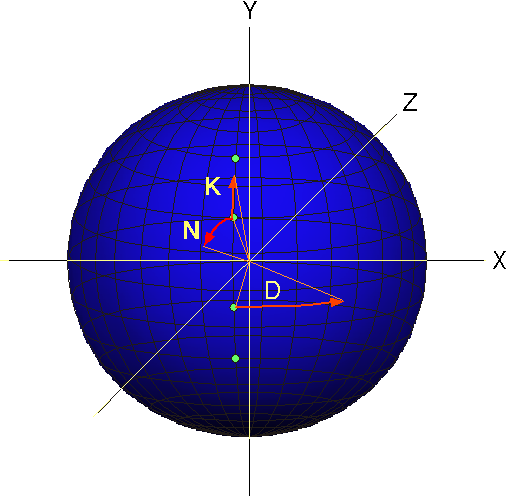Section 7Spreading the input data ( D ) moves a point on the surface of the sphere along the X and Z axis ( changes the longitude value ). Spreading the key ( K ) moves a point on the surface of the sphere along the Y and Z axis ( changes the latitude value ). Spreading the node ( N ) moves a point on the surface of the sphere along the X and Y axis ( changes the longitude and latitude values ) and appears as circular movement of the face of the sphere. The Edata is the vectors from the center to the points on the surface of the sphere. Since spreading is a mixture of fixed data ( input data ) and variable data ( noise ), with each encryption given the same data and key will produce varying Edata. Picking a random starting point in hyperspace ( any Vz vector ) also causes the Edata to vary.

Last updated: Mar 4, 2011

WXC